# please help 11. Disproportionation a. Consider the following reaction. 3Ch 5CL + Cls. Write two balanced half equati...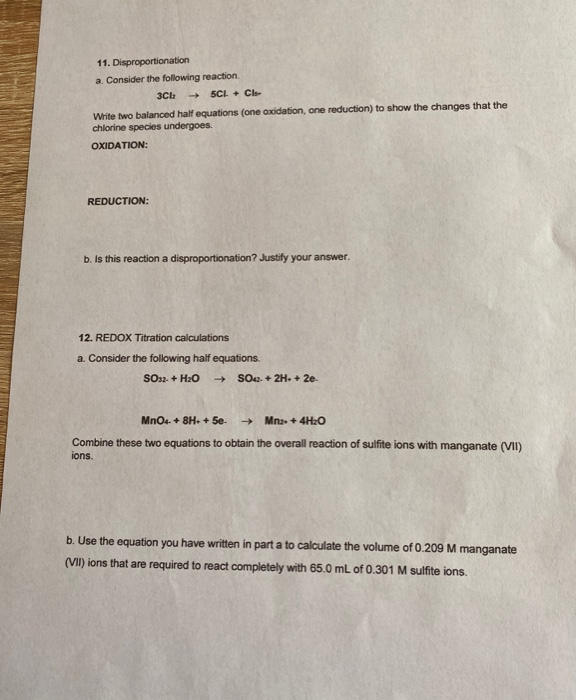11. Disproportionation a. Consider the following reaction. 3Ch 5CL + Cls. Write two balanced half equations (one oxidation, one reduction) to show the changes that the chlorine species undergoes. OXIDATION: REDUCTION: b. Is this reaction a disproportionation? Justify your answer. 12. REDOX Titration calculations a. Consider the following half equations. SO32- + H2O + SO42- + 2H+ + 2e. MnO4- + 8H. + 5e- → Mna: + 4H2O Combine these two equations to obtain the overall reaction of sulfite ions with manganate (VII) ions. b. Use the equation you have written in part a to calculate the volume of 0.209 M manganate (VII) ions that are required to react completely with 65.0 mL of 0.301 M sulfite ions.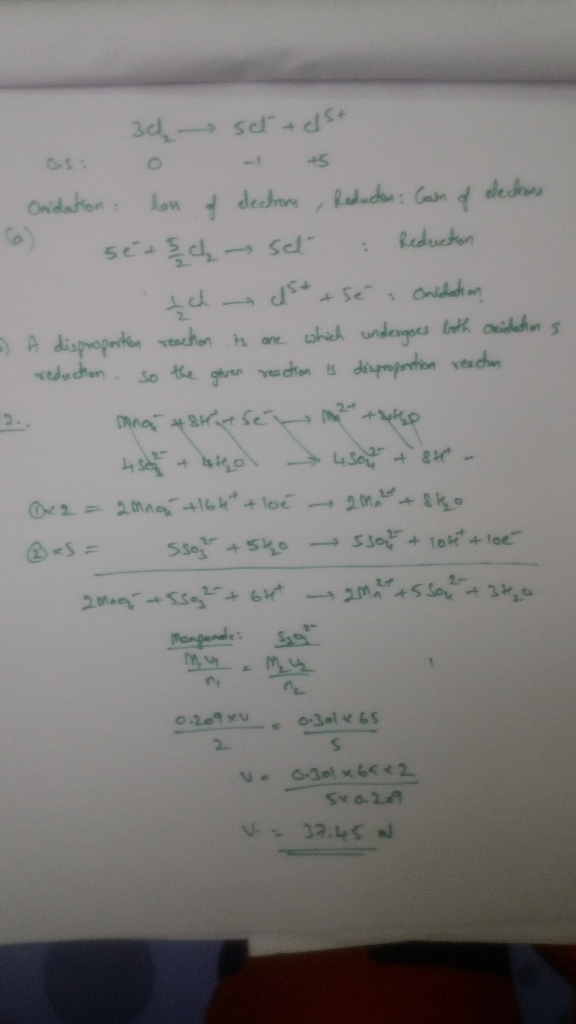Similar Homework Help Questions
• ### Write balanced half-reactions for the following redox reaction: reduction: 0 oxidation: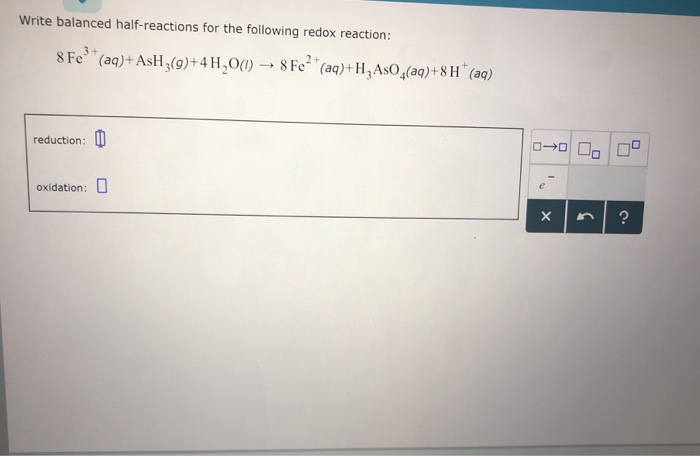Answer Write balanced half-reactions for the following redox reaction: reduction: 0 oxidation: Write balanced half-reactions for the following redox reaction: reduction: 0 oxidation:

• ### Enter electrons as e. The following skeletal oxidation-reduction reaction occurs under acidic conditions. Write...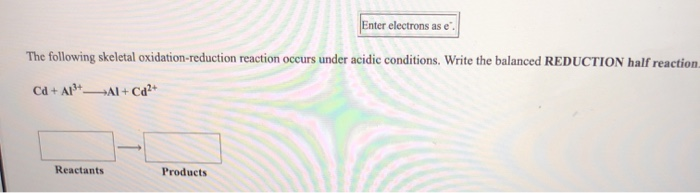Enter electrons as e. The following skeletal oxidation-reduction reaction occurs under acidic conditions. Write the balanced REDUCTION half reaction Cd + A13+ Al + Cd2+ Reactants Products Use the References to access important values if needed for this question er electrons as e skeletal oxidation-reduction reaction occurs under acidic conditions. Write the balanced REDUCTION half reaction Products Reactants Use the References to access important values if needed for this question. Enter electrons as e The following skeletal oxidation-reduction reaction occurs...

• ### write the balanced oxidation half-reaction for this cell...

Consider the following unbalanced redox reaction which is being used to run a voltaic cell:Cr(s) + Fe2+(aq)------> Cr3+(aq) + Fe(s)a. write the balanced oxidation half-reaction for this cell.b. write the balanced reduction half-reaction for this cell.c. write a balanced chemical equation that describes the overall cell reaction.d. assuming that the cell is operating at standard conditions, what would be the potential of the cell in volts?e. if [Cr3+] = 0.00010 M and [Fe2+] = 2.0 M, what would be the...

• ### Study this chemical reaction: FeI2(aq) +Mg(s)→  MgI2(aq) +Fe(s) Then, write balanced half-reactions describing...

Study this chemical reaction: FeI2(aq) +Mg(s)→  MgI2(aq) +Fe(s) Then, write balanced half-reactions describing the oxidation and reduction that happen in this reaction. Oxidation: Reduction:

• ### 2. Label each element with its oxidation state, use arrows to identify the reduction and oxidation, and balance the following redox reaction, which takes place under basic conditions. Please draw a b...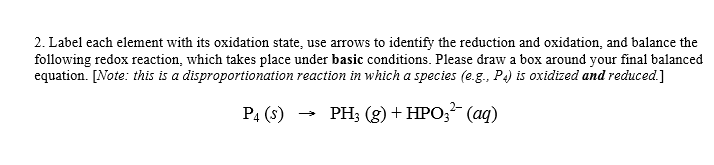2. Label each element with its oxidation state, use arrows to identify the reduction and oxidation, and balance the following redox reaction, which takes place under basic conditions. Please draw a box around your final balanced equation. [Note: this is a disproportionation reaction in which a species (e.g., P is oxidized and reduced.] P4 (s)PH (g) +HPO,2(aq) 2. Label each element with its oxidation state, use arrows to identify the reduction and oxidation, and balance the following redox reaction, which...

• ### 9. What is the coefficient in front of H' when the half-reaction is correctly balanced in acidic solution (with low...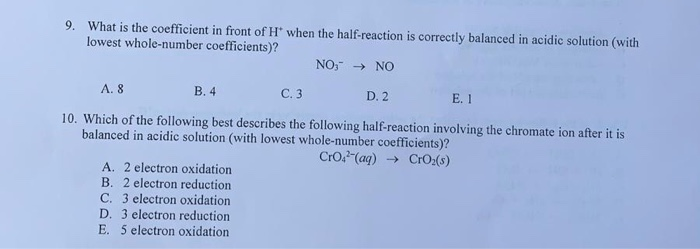9. What is the coefficient in front of H' when the half-reaction is correctly balanced in acidic solution (with lowest whole-number coefficients)? NO," → NO A. 8 B.4 - C.3 D. 2 E. I 10. Which of the following best describes the following half-reaction involving the chromate ion after it is balanced in acidic solution (with lowest whole-number coefficients)? CrO2-(aq) → CrO2(8) A. 2 electron oxidation B. 2 electron reduction C. 3 electron oxidation D. 3 electron reduction E. 5...

• ### Question 1: Consider the following oxidation-reduction reaction: I−(aq)+Cu+(aq)→IO4−(aq)+Cu(s) A: What are the initial a...

Question 1: Consider the following oxidation-reduction reaction: I−(aq)+Cu+(aq)→IO4−(aq)+Cu(s) A: What are the initial and final oxidation states of iodine? B: What are the initial and final oxidation states of copper? C: What element is reduced? D:What is the reducing agent? E: What element is oxidized? F: What is the oxidizing agent? G: Give the atom- and electron-balanced oxidation half-reaction occurring in acidic solution. Express your answer as a chemical equation. Include all phases. H: Give the atom- and electron-balanced reduction...

• ### Balance the following oxidation-reduction reactions using the half-reaction method. 1. HCOOH (aq) + MnO.. (aq) → CO2 (g) + Mn2. Acidie solution Identify the reduction half Identify the oxidation...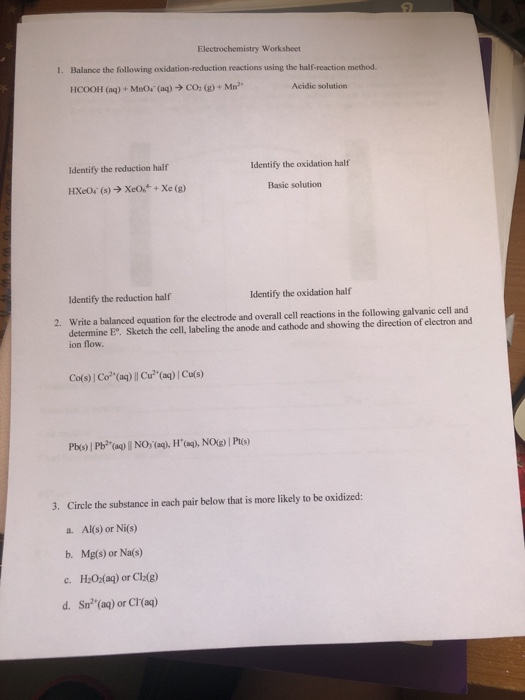Balance the following oxidation-reduction reactions using the half-reaction method. 1. HCOOH (aq) + MnO.. (aq) → CO2 (g) + Mn2. Acidie solution Identify the reduction half Identify the oxidation half Basic solution Identify the reduction half Identify the oxidation half Write a balanced equation for the electrode and overall cell reactions in the following galvanic cell and determine E°. Sketch the cell, labeling the anode and cathode and showing the direction of electron and ion flow. 2. 3. Circle the...

• ### When the following half reaction is balanced under basic conditions, what are the coefficients of the...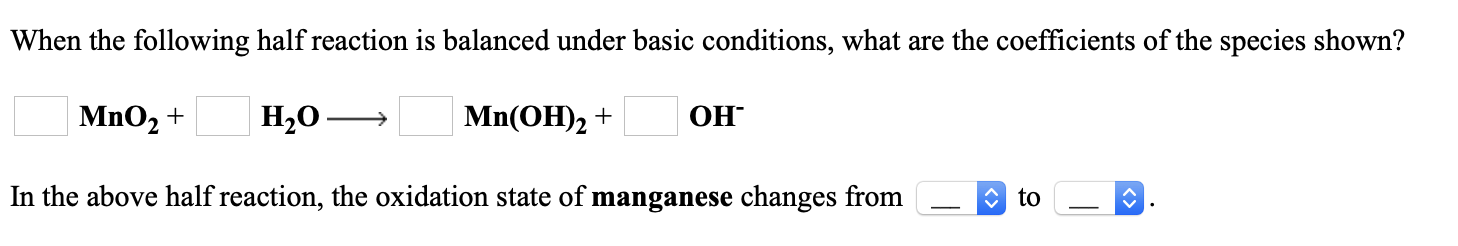When the following half reaction is balanced under basic conditions, what are the coefficients of the species shown? Но ОН MnO2 Mn(ОН)2 + In the above half reaction, the oxidation state of manganese changes from to

• ### whrite the balanced oxidation half reaction and the balanced reduction half reaction for this Il SuicS TIN OLI COLL...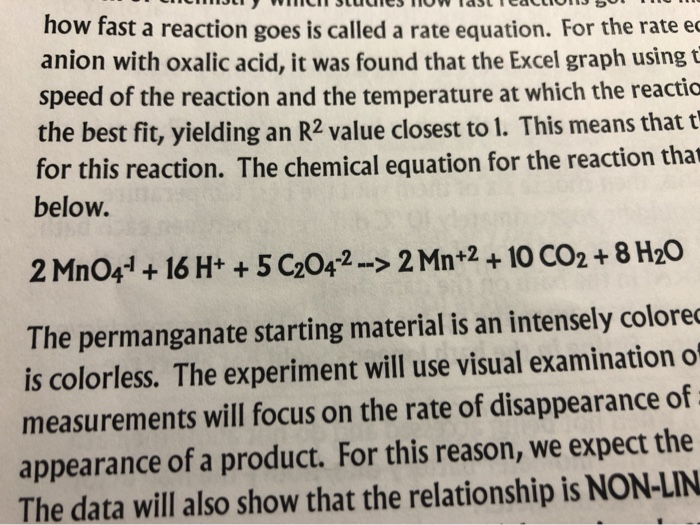whrite the balanced oxidation half reaction and the balanced reduction half reaction for this Il SuicS TIN OLI COLLIDE 111) " how fast a reaction goes is called a rate equation. For the rate € anion with oxalic acid, it was found that the Excel graph using speed of the reaction and the temperature at which the reactic the best fit, yielding an R2 value closest to 1. This means that t for this reaction. The chemical equation for the...

Need Online Homework Help?